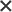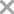0 / 300

## ￥128   2018年开始顽固性腹泻，除了馒头和粥，其他任何东西不能吃，吃了一点就一天腹泻3到4次。西医和中医都有看过，中药服用无效果，益生菌之类的都没有用。20年前胆囊切除，现胆管有小结石。就医两年多，人越来越瘦，加之营养不良，贫血。 也是两年前经常和子女吵架，背后辱骂，认为生病都是由子女害的，是否有心理问题？是否有必要去心理科就诊。现在腹泻看了很多医院，住院2次全身检查，肠镜检查，都未能使腹泻症状痊愈。请求医生帮助暂无内容

|

•妇科

•男科

•耳鼻咽喉科

•皮肤科

• 骨科
• 儿科
• 妇产科
• 口腔科
• 神经内科
• 泌尿外科
• 神经外科
• 乳腺外科
• 眼科
• 普外科
• 感染科
• 整形外科
• 外科
• 心血管内科
• 消化内科
• 呼吸内科

•王涛 主治医师 在线

河北医科大学第一医院 肾内科

• 痛风和高尿酸血症
• IgA肾病
• IGA
• 高血压肾损害
• 早泄
• 风湿类风湿性关节炎
• 肾囊肿
• 慢性肾功能衰竭
• 慢性肾盂肾炎
• 肾结石
• 勃起障碍
• 肾炎
• 腰痛
• 痛风
• 小儿原发性肾病综合征
• 慢性间质性肾炎
• 肾积水
• 狼疮性肾炎
• 妇科炎症
• 膜性肾病
• 干燥综合征
• 肾小球肾炎
• 慢性肾脏疾病
• 前列腺炎
• 过敏性紫癜
• 过敏性紫癜性肾炎
• 系统性红斑狼疮
• 感染
• 风湿
• 其他证
• 糖尿病肾病
• 蛋白尿
• 痛风性肾病
• 尿道感染
• 肾小管酸中毒
• 双肾
• 尿崩症
• 肾病综合征
• 带状疱疹
• 肾病
• 肾移植状态

1574个回答 ·100%回答率 ￥12 问TA

•蒋卫民 主任医师 在线

复旦大学附属华山医院 感染发热

• 发热待查
• 血吸虫病
• 颅内炎症
• 肺外结核
• 克雷白杆菌属感染
• 病毒性肝炎
• 寄生虫性尿路感染
• 慢性乙型肝炎
• 结核性脑膜炎
• 艾滋病
• 肝炎
• 沙门氏菌肠道感染
• 金黄色葡萄球菌感染
• 肺炎
• 乙型病毒性肝炎
• 多个部位的其他急性上呼吸道感染
• 多肌炎
• 肝硬化
• 发热
• 腰椎结核
• 阿米巴性痢疾
• 乙肝
• 动脉溃疡
• 支气管炎
• 丙型肝炎
• 流感
• 脂肪肝
• 脑膜炎
• 胸膜炎
• 丙型病毒性肝炎
• 感染
• 支原体感染
• 放射性肺炎
• 寄生虫病
• 梅毒
• 肺气肿
• 药物性肝损害
• 慢性活动性乙型肝炎
• 性病
• 化脓性脑膜炎
• 淋巴结核
• 戊肝
• 老年人结核性脑膜炎
• 其他特指的细菌感染
• 弓形虫病
• 低热症

623个回答 ·97%回答率 ￥68 问TA

•顾军花 主任医师 在线

上海中医药大学附属龙华医院 风湿病科

• 产后身痛病
• 大动脉炎
• 红斑狼疮
• 酒渣鼻
• 皮肌炎
• 结缔组织痣
• 银屑病关节炎
• 痛风
• 骨髓增生异常综合症
• 多肌炎
• 干燥综合征
• 风湿痛症
• 结缔组织病
• 桥本氏病
• 成人斯蒂尔病
• 发热
• 过敏性紫癜
• 产后身痛
• 系统性红斑狼疮
• 网状青斑
• 混合性结缔组织病
• 白塞病
• 腺样体肥大
• 硬皮病
• 贫血
• 风湿病
• 皮肤干燥症
• 风湿性关节炎
• 强直性脊柱炎
• 风湿类风湿性关节炎
• 便秘
• 失眠
• 痛风性关节炎
• 类风湿性关节炎
• 颈椎病
• 狼疮肾炎
• 结节性红斑
• 口腔溃疡
• 风湿性多肌痛

322个回答 ·100%回答率 ￥30 问TA

•姜伟 主任医师 在线

复旦大学附属妇产科医院杨浦院区 妇科门诊

• 产后子宫复旧不全
• 手术后恢复期
• 子宫息肉
• 人流术后残留
• 异常子宫出血
• 畸胎瘤
• 早期宫颈癌
• 滴虫性阴道炎
• 卵巢肿块
• 卵巢交界性肿瘤
• 子宫内膜炎
• 宫颈炎
• 感染
• 内分泌失调
• 输卵管积水
• 卵巢子宫内膜异位囊肿
• 子宫颈糜烂
• 卵巢妊娠
• 胎停育
• 异位妊娠
• 输卵管癌
• 月经过少病
• 功能性子宫出血
• 多胞胎
• 卵巢黏液性肿瘤
• 阴道癌前病变
• 盆腔炎
• 卵巢早衰
• 子宫憩室
• 继发闭经
• 老年性阴道炎
• 复发性卵巢癌
• 月经过多
• 月经失调
• 月经不规则
• 外阴癌
• 宫颈息肉
• 尖锐湿疣
• 避孕问题
• 阴道癌
• 子宫内膜异位
• 腹部肿物
• 支原体感染
• 产后出血
• 更年期月经过多
• 透明细胞癌
• 痛经
• 尿道炎
• 子宫内膜增生
• 先兆流产
• 妇科检查
• 人乳头瘤病毒感染
• 盆腔肿物
• 妇科炎症
• 宫颈囊肿
• 子宫纵隔
• 宫颈微癌
• 附件炎
• 腹壁子宫内膜异位
• 卵巢肿瘤
• 宫颈腺癌
• 闭经
• 子宫肉瘤
• 腺肌瘤
• 宫颈恶性肿瘤
• 念珠菌性外阴阴道炎
• 子宫脱垂
• 会阴侧切伤口裂开
• 妇科肿瘤
• 巧克力囊肿
• Ⅱ级宫颈上皮内肿瘤[CIN]
• 腹腔畸胎瘤
• 宫颈癌前病变
• 子宫平滑肌瘤
• 经表皮排除疾患
• 乳房恶性肿瘤
• 阴道炎
• 宫颈原位癌
• 外阴息肉
• 卵巢囊肿
• 自发流产
• 巴氏腺囊肿
• 葡萄胎
• 卵巢纤维瘤
• 绝经后子宫内膜癌
• 流产
• 子宫附件肿块
• 卵巢癌肉瘤
• 子宫内膜不典型增生
• 子宫内膜间质肉瘤
• 慢性子宫颈炎
• 出血
• 盆腔囊肿
• 乳腺结节
• 多囊卵巢综合症
• 宫颈疾病
• 妊娠合并子宫肌瘤
• 卵巢明细胞肿瘤
• 子宫内膜癌
• 卵巢癌
• 绝经期出血
• 宫腔粘连
• 子宫腺肌病
• 盆腔包块
• 更年期综合征
• 乳房小叶增生
• 附件肿物
• 无排卵型功能失调性子宫出血
• 宫颈HPV感染
• 卵巢恶性肿瘤
• 尿道感染
• 人工流产
• 输卵管积液
• 滋养层细胞瘤
• 盆腔积液
• 宫颈上皮内瘤变
• 输卵管堵塞

165个回答 ·93%回答率 ￥30 问TA

•马勇 副主任医师 在线

上海交通大学医学院附属第九人民医院（漠河路院区） 皮肤科

• 神经性皮炎
• 胶状粟粒疹
• 软瘊
• 白癜风
• 冻疮
• 小儿血管瘤
• 老年斑
• 毛细血管扩张
• 蓝痣
• 牛奶斑
• 鲜红斑痣
• 咖啡牛奶斑
• 丘疹
• 雀斑
• 黄褐斑
• 皮下囊肿
• 胎记
• 毛周角化病
• 化脓性肉芽肿
• 病毒疹
• 咖啡斑
• 脂瘤
• 瘢痕疙瘩
• 灰指甲
• 贝克痣
• 皮炎
• 额部皮肤恶性肿瘤
• 疤痕增生
• 晒斑
• 疣状痣
• 褐青色痣
• 白斑[白癜风]
• 荨麻疹
• 红血丝
• 银屑病
• 单纯性雀斑样痣
• 扁平疣
• 伊藤痣
• 皮疹
• 皮肤过敏
• 感染
• 冻伤
• 单纯疱疹
• 色素痣
• 疤痕
• 玫瑰痤疮
• 面部擦伤
• 血管瘤
• 皮脂腺痣
• 毛发上皮瘤
• 激光治疗术后状态
• 痘样痤疮
• 股癣
• 带状疱疹
• 隆突性皮肤纤维肉瘤
• 化脓性汗腺炎
• 疣病
• 汗管瘤
• 色素失调症
• 太田痣
• 纹身

119个回答 ·100%回答率 ￥30 问TA49个回答 · 56%回答率0 / 3001、请您尽可能完善问题描述，以便医生能更全面细致地提供专业指导意见；

2、若问题提出后超出48小时无人回答，系统将自动退款；问题提出后一经医生回答，提问金将冻结，在问题完成时结算给医生，基于此情况下平台不予退款；

3、非定向提问（即一问多答形式），您可在问题提出72小时内，可对每一位医生的回答进行【有帮助】及【没帮助】评价，与平台一起不断提升医生的服务质量，在问题持续的48小时内随时可评价，评价不会影响问题状态；

4、定向提问（即提问某个指定医生），该医生首次回复24小时内，您可点击“免费问一次”进行追问；追问功能为医生额外提供的爱心服务，也可能存在不回答的情况；

5、若您提问内容涉及违反平台规范或相关法律法规，将作下线处理；

6、请勿在提问内容中提供或展示个人隐私信息，包含但不限于个人姓名、联系方式、住址等；

7、您在此确认并同意，微医有权对您在平台发布的公开的信息享有免费的、永久性的、不可撤销的、非独家的和完全再许可的权利，并许可微医使用、复制、改编、公开展示此等内容；

8、微医非常注重对您个人隐私的保护。我们将使用安全技术和相关程序保护您的个人信息不被未经授权的访问、使用或泄露；

9、医生回答仅供用户参考，不能被认为是医嘱、诊断证明或其他类似性质的文件；

10、医生回答内容不代表本平台观点，对您在本平台之外与特定或不特定医生所进行的任何接洽行为之后果，不承担责任；